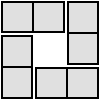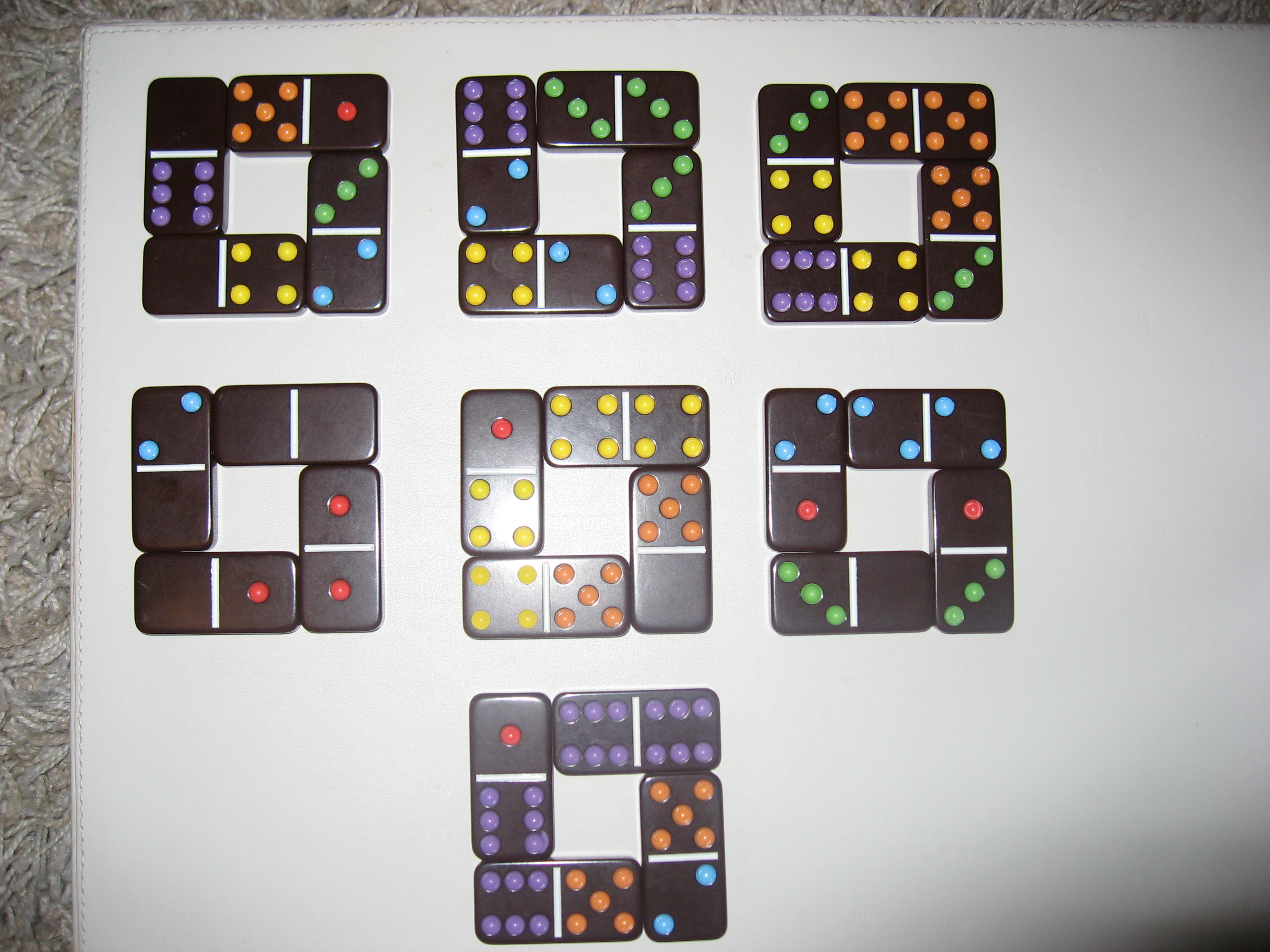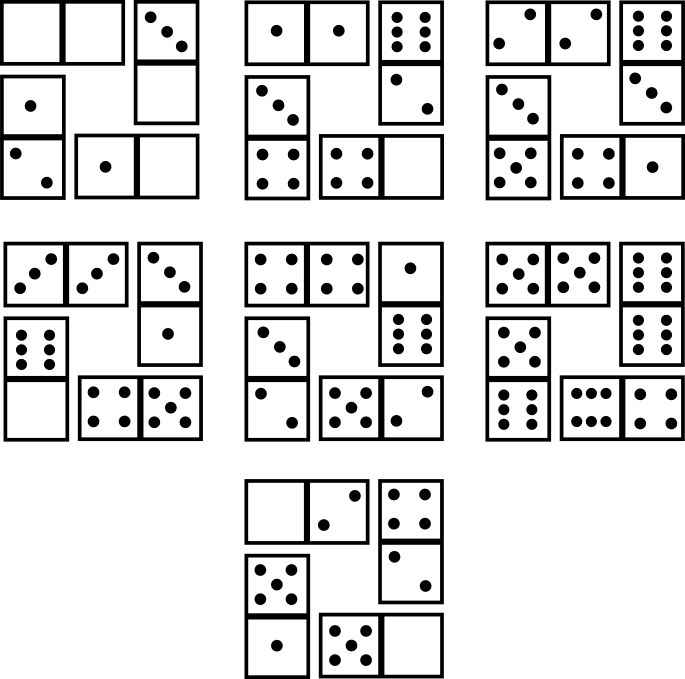#### You may also like### Domino Square

Use the 'double-3 down' dominoes to make a square so that each side has eight dots.### 4 Dom

Use these four dominoes to make a square that has the same number of dots on each side.### Eight Dominoes

Using the 8 dominoes make a square where each of the columns and rows adds up to 8

# Dominoes

##### Age 7 to 16 Challenge Level:

Here are two possible solutions to the "windows" problem: